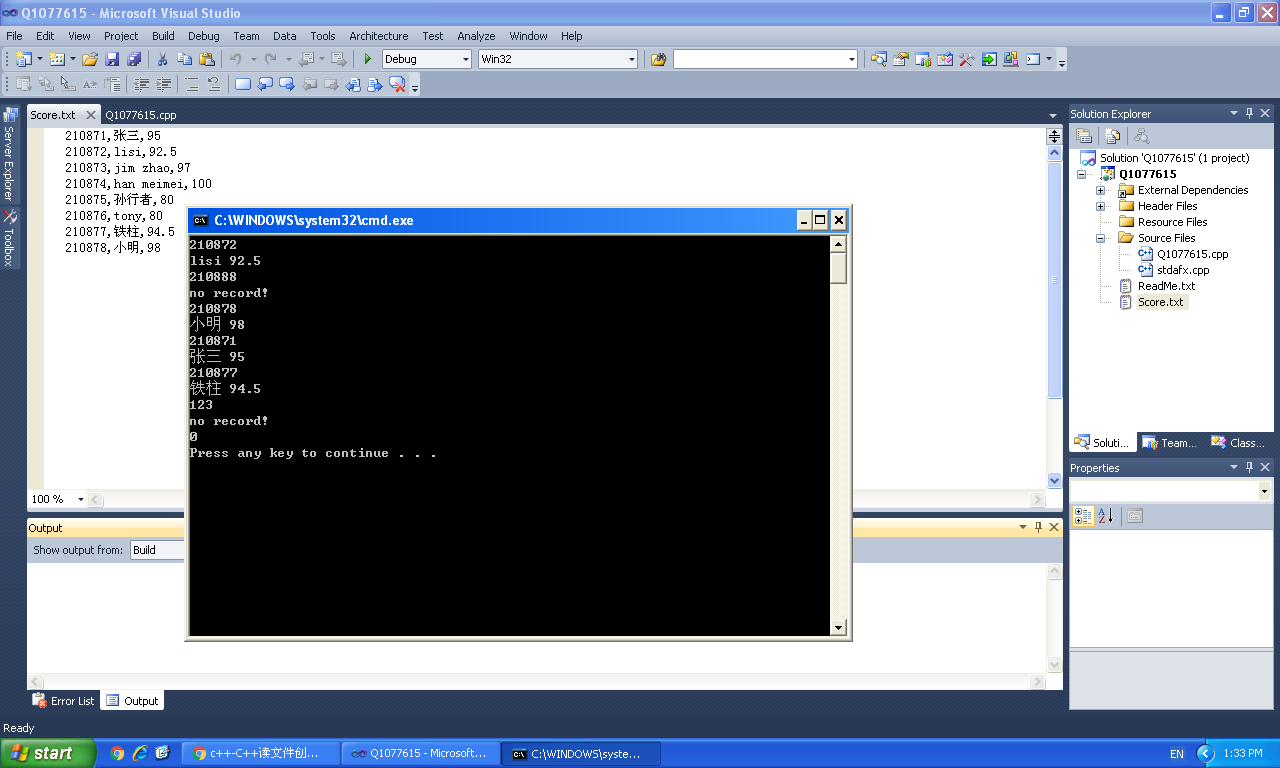C++读文件创建链表问题

C++期末成绩存放在文本文件 “Score.txt”中，要求编写程序读取该文本文件创建链表，并在链表上根据学号查找姓名和成绩。

文本文件的各列之间使用空格分隔，文件行数不确定。
要求使用ifstream类和ofstream类的对象读取和写入数据。
链表的一个结点可声明如下：
struct student
{
unsigned long id;
string name;
float score;
student *next; //下一个结点的指针
}
循环从键盘读入学号，查询对应的姓名和成绩，输入学号为0时退出循环。

1个回答

# 如果问题得到解决，请点下采纳

// Q1077615.cpp : Defines the entry point for the console application.
//

#include <stdio.h>
#include <stdlib.h>
#include <iostream>
#include <string>
using namespace std;
struct student
{
unsigned long id;
string name;
float score;
student *next; //下一个结点的指针
};

int main()
{
FILE * fp = fopen("Score.txt", "r+");
while (true)
{
unsigned long id;
char name;
float score;
if (fscanf(fp, "%ld,%[^,],%f", &id, name, &score) == EOF) break;
p->next = new student;
p = p->next;
p->next = NULL;
p->id = id;
p->name = name;
p->score = score;
}
int n;
while (1)
{
cin >> n;
if (n == 0) break;
while (p)
{
if (p->id == n)
{
cout << p->name << " " << p->score << endl;
break;
}
p = p->next;
}
if (!p || p->id != n)
cout << "no record!" << endl;
}
return 0;
}


210871,张三,95
210872,lisi,92.5
210873,jim zhao,97
210874,han meimei,100
210875,孙行者,80
210876,tony,80
210877,铁柱,94.5
210878,小明,98